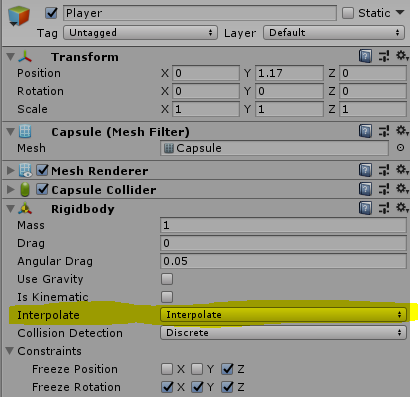I’m working on a first person controller and i’m using Rigidbody to make the movement for the controller.

Altough i notice that when i move my character around on a simple plane, it starts skipping(jumping). I don’t want to freeze the Y position, because i want my character to be jumping, and go up and down hills ect. Anyone have any tips on making this skipping disappear?

``````Direction = new Vector3(0,0,0);
if (Input.GetKey(KeyCode.W))
{
Direction += Vector3.forward;
}
if (Input.GetKey(KeyCode.A))
{
Direction += Vector3.left;
}
if (Input.GetKey(KeyCode.D))
{
Direction += Vector3.right;
}
if (Input.GetKey(KeyCode.S))
{
Direction += Vector3.back;
}
if (Input.GetKey(KeyCode.Space) && CurrentlyJumping == false)
{
CurrentlyJumping = true;
}
CharSpeed = InputSpeed;
Direction.Normalize();
``````

This is the function i’m calling for movement.

Gravity is on by the way

Turn on interpolation on your player’s rigidbody.Check to see if you have more than one collider active on the object - if you’ve mistakenly done this, the object will push itself / jerk in unintended directions.

To fix the skipping, what people usually do is lower the gravity when the character ‘is grounded’ (has ground under his feet). For this to work, first the gravity on the rigidbody has to be disabled. Second, gravity has to be applied manually.

If you want to make the controller less slidy, play with the drag/speed settings to get the desired result.Also, make sure to always execute additive Physics functions in the FixedUpdate() function:

``````var Direction : Vector3 = Vector3.zero;
var Speed : float = 5;
var JumpStrength : float = 5;
var HalfCharacterHeight : float = 1;
var DefaultGravity : float = 9.81;
var GroundedGravity : float = 0.1;
private var gravity : float = 9.81;
private var isGrounded : boolean = false;

function Update () {

//we cast a ray downwards to the characters feet, to check if he's grounded
isGrounded = Physics.Raycast(transform.position, -transform.up, HalfCharacterHeight);

//change the gravity setting to Default/Grounded gravity based on the result of the raycast
if(isGrounded) gravity = GroundedGravity;
else gravity = DefaultGravity;

Direction = Vector3.zero;

//I'm using unity's input manager instead. You can change this in Edit/P.Settings/Input
Direction.x = Input.GetAxis("Horizontal"); //you can also change this to
Direction.z = Input.GetAxis( "Vertical" ); //GetAxisRaw

if(Direction.magnitude > 0) //if the direction isn't zero normalize it
Direction = Direction.normalized; //so that diagonal movement isn't faster

//if the space key was pressed and the character is grounded, jump!
if(Input.GetKeyDown(KeyCode.Space) && isGrounded) {
//using Impulse, to make the jump more realistic
}
}

function FixedUpdate () {

if(Direction.magnitude > 0) //if the direction isn't zero add force

}
``````

You could also try changing the Physics(Fixed) timestep in the Edit/Project Settings/Time menu. This can also solve fast rigidbodies flying trough walls.—David

Well i guess it’s a little embarrassing but i solved the skipping problem by just changing the way i move. I stopped using Rigidbody and simply used transform.translate. With a little help of the nice people answering this question i solved it!

This is the code.

``````float h = Input.GetAxis("Horizontal");
Vector3 Direction = new Vector3(h, 0, 0);
float CharSpeed = InputSpeed;

if (Input.GetKey(KeyCode.W))
{
Direction += Vector3.forward;
}
if (Input.GetKey(KeyCode.S))
{
Direction += Vector3.back;
}
if (Input.GetKey(KeyCode.A))
{
Direction += Vector3.left;
}
if (Input.GetKey(KeyCode.D))
{
Direction += Vector3.right;
}
Direction.Normalize();
transform.Translate(Direction * CharSpeed);
``````

I’m still using Rigidbody for gravity and ect, but the reason why i used AddForce from the start was because i was having problems with the movement adjusting to the camera angle.
Hope this will help anyone else who’s having similiar problems.

You might want to try changing your inputs to:

`float h = input.getAxis(“Horizontal”);

float v = input.getAxis(“Vertical”);

direction = (h, v, 0);`

When you add force with a getbuttondown the full amount of the force is added immediately,
but the axis is a little smoother.